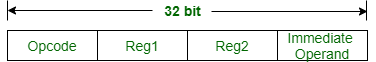Related Articles

# ISRO | ISRO CS 2020 | Question 7

• Last Updated : 10 May, 2020

Consider a 32-bit processor which supports 70 instructions. Each instruction is 32 bit long and has 4 fields namely opcode, two register identifiers and an immediate operand of unsigned integer type. Maximum value of the immediate operand that can be supported by the processor is 8191. How many registers the processor has ?
(A) 32
(B) 64
(C) 128
(D) 16

Explanation: Given, 32-bit processor,Number of bits required for Opcode = ceiling(log2(70)) = 7

Number of bits required for Immediate operands = ceiling(log2(8191)) = ceiling (12.99) = 13

Now, Number of bits left to represents register bits = 32 – (7+13) = 12.

Since there are two register operands (i.e., Reg1 and Reg2), so 6 bits for each register.

Hence, number of registers = 26 = 64 registers.

Option (B) is correct.

Quiz of this Question

My Personal Notes arrow_drop_up